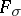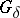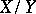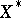# Asplund space

(diff) ← Older revision | Latest revision (diff) | Newer revision → (diff)

strong differentiability space

A Banach spacesuch that every continuous convex function on an open convex subsetofis Fréchet differentiable at the points of a dense-subset of(cf. also Convex function (of a real variable); Fréchet derivative; set of type()). Such a space mirrors the differentiability properties of continuous convex functions on Euclidean space. These spaces were originally called strong differentiability spaces by E. Asplund [a1], who began serious investigation of them. I. Namioka and R.R. Phelps [a2] and C. Stegall [a3] established the significance of this class of spaces by proving that a Banach spaceis an Asplund space if and only if its dual(cf. also Adjoint space) has the Radon–Nikodym property. The most useful characterization is that a Banach spaceis an Asplund space if and only if every separable subspace has a separable dual (cf. also Separable space).

The class of Asplund spaces has many stability properties: the class is closed under topological isomorphisms, closed subspaces of Asplund spaces are Asplund, quotients of Asplund spaces are Asplund; furthermore, the class has the three-space property, that is, if a Banach spacehas a closed subspacewhich is Asplund and the quotient spaceis Asplund, thenis Asplund [a2].

A Banach space which has an equivalent norm that is Fréchet differentiable away from the origin is an Asplund space [a4]; however, R. Haydon [a5] has given an example of an Asplund space not having an equivalent norm that is Gâteaux differentiable away from the origin (cf. also Gâteaux derivative).

A significant property of Asplund spaces, and with application in optimization theory, was established by D. Preiss [a6], who showed that every locally Lipschitz function (cf. also Lipschitz condition) on an open subset of an Asplund space is Fréchet differentiable at the points of a dense subset of its domain.

An Asplund space can be characterized by geometrical properties of its dual. A Banach spaceis an Asplund space if and only if every non-empty bounded subset of its dualhas weak--slices of arbitrarily small diameter. This property can be used to show that any minimal weak-upper semi-continuous weak-compact and convex-valued set-valued mapping on an open subset of an Asplund space is residually single-valued. It also provides the characterization that a Banach spaceis an Asplund space if and only if every non-empty weak-compact convex subset of the dualis the weak-closed convex hull of its weak-strongly exposed points, [a2] (cf. also Weak topology). R.E. Huff and P.D. Morris [a7] showed that this property is equivalent to every bounded closed convex subset of the dualbeing the closed convex hull of its extreme points.

There has been a less productive investigation of the larger class of weak Asplund spaces, called weak differentiability spaces by E. Asplund, where every continuous convex function on an open convex subset is Gâteaux differentiable at the points of a dense-subset of the domain [a8]. More amenable is the subclass of Asplund-generated spaces, that is, Banach spaces which contain, as a dense subspace, the continuous linear image of an Asplund space.

How to Cite This Entry:
Asplund space. Encyclopedia of Mathematics. URL: http://encyclopediaofmath.org/index.php?title=Asplund_space&oldid=16665
This article was adapted from an original article by J.R. Giles (originator), which appeared in Encyclopedia of Mathematics - ISBN 1402006098. See original article